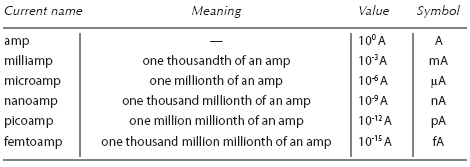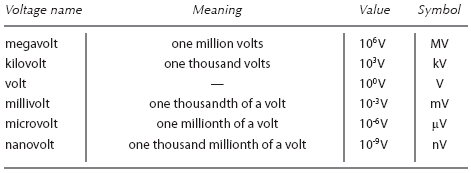# Electronic componentsElectronics guide > The very first steps > Electronic components

The fact that different resistances produce different currents if a voltage is applied across them, or produce different voltages if a current is applied through them, is one of the most useful facts in electronics.

In electronics, an amp of current is very large — usually we only use much smaller currents, say, a thousandth or so of an amp. Sometimes we even use currents smaller than this, say, a millionth of an amp! Similarly, we sometimes need only small voltages, too.

Resistances are extremely useful in these cases, because they can be used to reduce the current flow or the voltage produced across them, due to the effects of Ohm’s law. We’ll look at ways and means of doing this in the next chapter. All we need to know for now is that resistances are used in electronics to control current and voltage.

Table 1.1 shows how amps are related to the smaller values of current. A thousandth of an amp is known as a milliamp (unit: mA). A millionth of an amp is a microamp (unit: μA).Table 1.1 Comparing amps with smaller values of current

Even smaller values of current are possible: a thousand millionth of an amp is a nanoamp (unit: nA); a million millionth is a picoamp (unit: pA). Chances are, you will never use or even specify a current value smaller than these, and you will rarely even use picoamp. Milliamps and microamps are quite commonly used, though.

It’s easy to move from one current value range to another, simply by moving the decimal point one way or the other by the correct multiple of three decimal places. In this way, a current of 0.01 mA is the same as a current of 10 μA which is the same as a current of 10,000 nA and so on.

Table 1.2 shows, similarly, how volts are related to smaller values of voltage. Sometimes, however, large voltages exist (not so much in electronics, but in power electricity) and so these have been included in the table. The smaller values correspond to those of current, that is, a thousandth of a volt is a millivolt (unit: mV), a millionth of a volt is a microvolt (unit: μV) and so on — although anything smaller than a millivolt is, again, only rarely used.Table 1.2 Comparing volts with smaller and larger voltages

Larger values of voltage are the kilovolt, that is, one thousand volts (unit: kV) and the megavolt, that is, one million volts (unit: MV). In electronics, however, these are never used.

<< Relationships